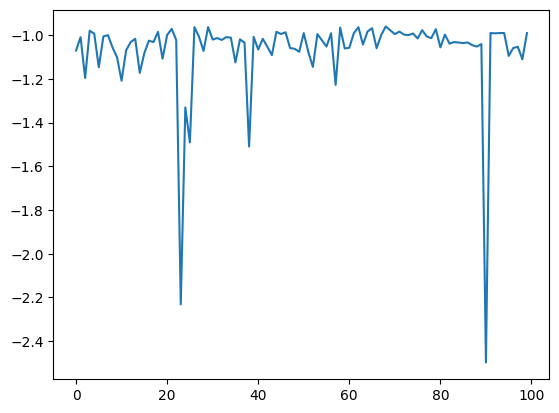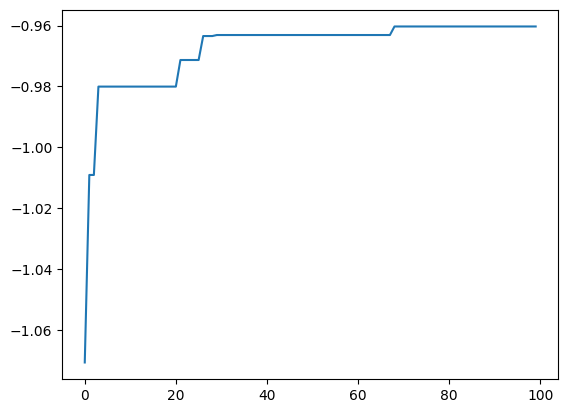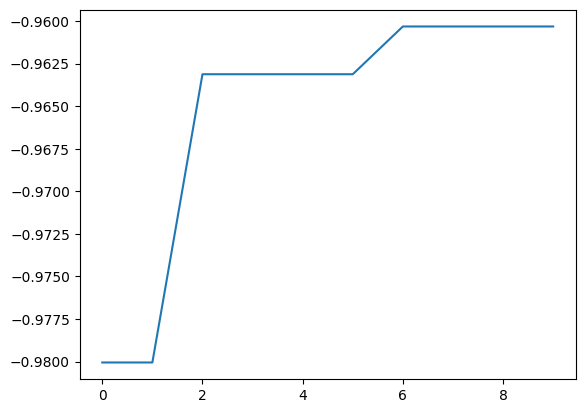# Search multiple candidates at once

This is a tutorial for evaluating two or more candidates at once in a single search step.

## Prepare the search candidate data

As the previous tutorials, save the dataset file s5-210.csv into the subdirectory data, and load dataset from this file as the following:

:

import physbo

import numpy as np
import matplotlib.pyplot as plt
%matplotlib inline

X = A[:,0:3]
t  = -A[:,3]
return X, t

X = physbo.misc.centering(X)


## Definition of simulator

If num_search_each_probe (described below) is set to 2 or more, action will be input as an array of action IDs.
Thus, define the simulator to return a list of evaluation values for each action ID.

The definitions in the basic tutorial and simulator are the same, but keep in mind that t is a numpy.array, and when action is an array, self.t[action] will also be an array.

:

class simulator:
def __init__( self ):

def __call__( self, action ):
return self.t[action]


Example of running the simulator

:

sim = simulator()
sim([1,12,123])

:

array([-1.01487066, -1.22884748, -1.05572838])


## Performing optimizations

:

# set policy
policy = physbo.search.discrete.policy(test_X=X)

# set seed
policy.set_seed( 0 )


num_search_each_probe allows you to specify the number of candidates to evaluate in each search step.

In the following example, the simulator will be evaluated 2 × 10 = 20 times by random search and 8 × 10 = 80 times by Bayesian optimization.

argument.

• max_num_probes: Number of search steps.

• num_search_each_probe: Number of candidates to evaluate at each search step.

[ ]:

res = policy.random_search(max_num_probes=2, num_search_each_probe=10, simulator=simulator())

res = policy.bayes_search(max_num_probes=8, num_search_each_probe=10, simulator=simulator(), score='EI',
interval=2, num_rand_basis=100)


## Checking results

:

plt.plot(res.fx[0:res.total_num_search])

:

[<matplotlib.lines.Line2D at 0x7f9950ec84f0>]:

best_fx, best_action = res.export_all_sequence_best_fx()
plt.plot(best_fx)

:

[<matplotlib.lines.Line2D at 0x7f9920ef7460>]With res.export_sequence_best_fx(), you can get the best value obtained at each step and the history of the action.

The difference between res.export_all_sequence_best_fx() and res.export_all_sequence_best_fx() is that the information is not for each evaluation of the simulator, but for each search step. In this case, the total number of steps is 10, and the number of evaluations is 100.

:

best_fx, best_action = res.export_sequence_best_fx()
plt.plot(best_fx)

:

[<matplotlib.lines.Line2D at 0x7f9930b43f10>]